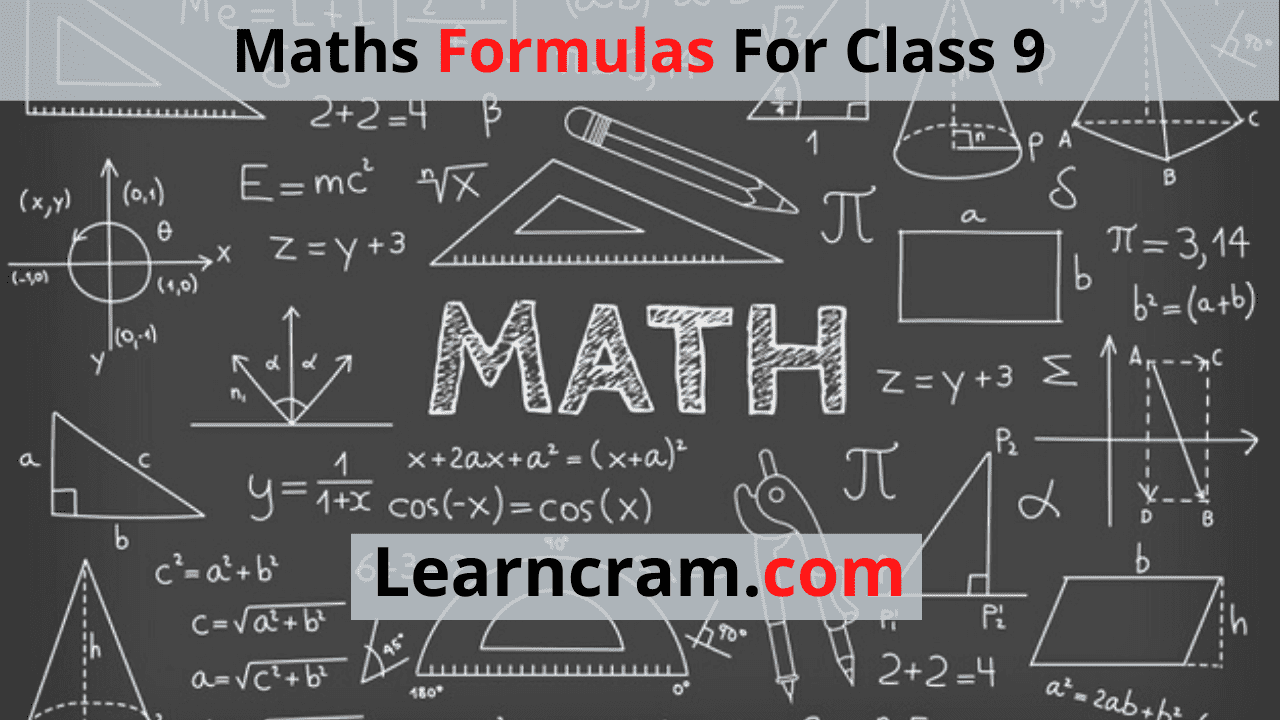# Maths Formulas For Class 9

For some Maths can be fun and for some, it can be a nightmare. Maths Formulas are difficult to memorise and we have curated a list of Maths Formulas for Class 9 just for you. You can use this as a go-to sheet whenever you want to prepare Class 9 Maths Formulas. Students can get Formulas on Algebra, Calculus, and Geometry that can be useful in your preparation and help you do your homework.

Here in this article, we have listed basic Maths formulas so that you can learn the fundamentals of Maths. Our unique way of solving Maths Problems will make you learn how the equation came into existence instead of memorizing it. Solve all the important problems and questions in Maths with the Best Maths Formulas for Class 9.

Feel free to directly use the best Maths formulas during your homework or exam preparation. You need to know the list of Class 9 formulas as they will not just be useful in your academic books but also in your day to day lives.

Remember the Maths Formulas in a smart way by making use of our list. You can practice Questions and Answers based on these Class 9 Maths Formulas. Students can get basic Maths formulas Free PDF Download for Class 9. Candidates can use the handy learning aid Maths Formulas PDF to have in depth knowledge on the subject as per the Latest CBSE Syllabus.

CBSE Class 9 Maths Formulas according to the Chapters are prepared by subject experts and you can rely on them during your preparation. Click on the topic you wish to prepare from the list of formulas prevailing.

## Class 9 Math Formula Tables

 Geometry Shapes Formulas for Class 9 Geometric Figure Area Perimeter Rectangle A= l × w P = 2 × (l+w ) Triangle A = (1⁄2) × b × h P = a + b + c Trapezoid A = (1⁄2) × h × (b1+ b2) P = a + b + c + d Parallelogram A = b × h P = 2 (b + h) Circle A = π r2 C = 2 π r
 Algebraic Identities For Class 9 (a+b)2=a22+2ab+b22 (a−b) 2=a2−2ab+b2 (a+b)(a–b)=a2–b2 (x+a)(x+b)=x2+(a+b)x+ab (x+a)(x–b)=x2+(a–b)x–ab (x–a)(x+b)=x2+(b–a)x–ab (x–a)(x–b)=x2–(a+b)x+ab (a+b) 3=a3+b3+3ab(a+b) (a–b)3=a33–b3–3ab(a–b) (x+y+z) 2=x2+y2+z2+2xy+2yz+2xz (x+y–z) 2=x2+y2+z2+2xy–2yz–2xz (x–y+z) 2=x2+y2+z2–2xy–2yz+2xz (x–y–z) 2=x2+y2+z2–2xy+2yz–2xz x3+y3+z3–3xyz=(x+y+z)(x2+y2+z2–xy–yz−xz) x2+y2=$$\frac {1}{2}$$ [(x+y) 2+(x–y) 2] (x+a)(x+b)(x+c)=x3+(a+b+c)x2+(ab+bc+ca)x+abc x3+y3=(x+y)(x2–xy+y2) x33–y3=(x–y)(x2+xy+y2) x2+y2+z2−xy–yz–zx=$$\frac {1}{2}$$ [(x−y) 2+(y−z) 2+(z−x) 2]<

### FAQs on Class 9 Maths Formulas

1. Where can I get Maths Formulas for Class 9?

You can find the list of all Maths Formulas pertaining to Class 9 from our page. In fact, all the formulas are arranged topic wise as per chapters and you can use them to score better grades in the exam.

2. How do I Learn Class 9 Maths Formulas?

Don’t try to mug up the formulas instead try finding the logic behind it so that it will be easy for you. However, there are some formulas that are hard to derive and you can memorize them. Practice as much as you can to understand the Maths Class 9 Formulas.

3. Is there a Website that provides all Maths formulas for Class 9?

Students can make use of our website to access all the Class 9 Maths Formulas as per the topics to make your learning process effective.

Final Words

We believe that the comprehensive list of basic Maths formulas for Class 9 will make your learning effective. You can simply click on the Topics to view the Class 9 Maths formulas and aid your preparation. If you feel any formula is missing that can be added to our list do drop us a comment and we will add it to the list.